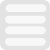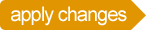## Learning SCL

The best way to learning to create animations with SCL is by experimenting with examples. You will find code examples on this site that allow you to edit the code to run it immediately.

## SCL Basics

create sprite from fan.png as spinner
where x=100 y=100 center=90,90
end
create routine as Start
launch spinner
run routine ThisWay
end
create routine as ThisWay
insert into spinner where
alt=(sub create rotation where speed=130
and distance=135 completion=ThatWay end)
end
create routine as ThatWay
insert into spinner where
alt=(sub create rotation where speed=-130
and distance=135 completion=ThisWay end)
end

## SCL code

Sprites
define images that are animated
Routines